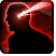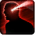Conditions

Related effects, buffs and debuffs

• [hidden] [0s]Effect #1

 Slot: EffectOther Duration: 0s Tick rate: does not tick # occurrences: 0 Tags: tag.​abl.​smuggler.​pistol_ability
• On Apply

Only when the following conditions are met:

• If TARGET is dual wielding, with weapon mode 3

Perform the following actions:

• Play appearance epp . smuggler . headshot_dual
• Invoke Projectile
- Determine Hit = (bool) false
- Timed Projectile = (bool) false
- Hit Checked = (bool) false
- Is Special Ability = (bool) false
- Ignore Dual Wield Modifier = (bool) false
- Slot = (int) 5
- Spell Type = (int) 1
- Travel Speed = (float) 4
- Travel Time = (time) 0s
• On Apply

Only when the following conditions are met:

• If TARGET is not dual wielding, with weapon mode 3

Perform the following actions:

• Play appearance epp . smuggler . headshot
• Invoke Projectile
- Determine Hit = (bool) false
- Timed Projectile = (bool) false
- Hit Checked = (bool) false
- Is Special Ability = (bool) false
- Ignore Dual Wield Modifier = (bool) false
- Slot = (int) 5
- Spell Type = (int) 1
- Travel Speed = (float) 4
- Travel Time = (time) 0s
• When projectile completed travel and hit target

Only when the following conditions are met:

• <
• If toughness of PRIMARY TARGET is <= Standard
AND
• If TARGET is dual wielding, with weapon mode 3
>

Perform the following actions:

• Weapon Damage
- Is Special Ability = (bool) true
- Ignore Dual Wield Modifier = (bool) true
- Flurry Blows Min = (int) 1
- Flurry Blows Max = (int) 1
- Threat Percent = (float) 0
- Standard Health Percent Max = (float) 0.288
- Standard Health Percent Min = (float) 0.288
- Amount Modifier Fixed Max = (float) 0
- Amount Modifier Fixed Min = (float) 0
- Amount Modifier Percent = (float) 0.92
- Coefficient = (float) 2.88
- Health Steal Percentage = (float) 0
- Slots = Int(2)
• Weapon Damage
- Is Special Ability = (bool) true
- Ignore Dual Wield Modifier = (bool) false
- Flurry Blows Min = (int) 1
- Flurry Blows Max = (int) 1
- Threat Percent = (float) 0
- Standard Health Percent Max = (float) 0
- Standard Health Percent Min = (float) 0
- Amount Modifier Fixed Max = (float) 0
- Amount Modifier Fixed Min = (float) 0
- Amount Modifier Percent = (float) 0.92
- Coefficient = (float) 0
- Health Steal Percentage = (float) 0
- Slots = Int(4)
• When projectile completed travel and hit target

Only when the following conditions are met:

• <
• If toughness of PRIMARY TARGET is <= Standard
AND
• If TARGET is not dual wielding, with weapon mode 3
>

Perform the following actions:

• Weapon Damage
- Is Special Ability = (bool) true
- Ignore Dual Wield Modifier = (bool) true
- Flurry Blows Min = (int) 1
- Flurry Blows Max = (int) 1
- Threat Percent = (float) 0
- Standard Health Percent Max = (float) 0.288
- Standard Health Percent Min = (float) 0.288
- Amount Modifier Fixed Max = (float) 0
- Amount Modifier Fixed Min = (float) 0
- Amount Modifier Percent = (float) 0.92
- Coefficient = (float) 2.88
- Health Steal Percentage = (float) 0
- Slots = Int(2)
• When projectile completed travel and hit target

Only when the following conditions are met:

• <
• If toughness of PRIMARY TARGET is = Strong
AND
• If TARGET is dual wielding, with weapon mode 3
>

Perform the following actions:

• Weapon Damage
- Is Special Ability = (bool) true
- Ignore Dual Wield Modifier = (bool) true
- Flurry Blows Min = (int) 1
- Flurry Blows Max = (int) 1
- Threat Percent = (float) 0
- Standard Health Percent Max = (float) 0.197
- Standard Health Percent Min = (float) 0.197
- Amount Modifier Fixed Max = (float) 0
- Amount Modifier Fixed Min = (float) 0
- Amount Modifier Percent = (float) 0.31
- Coefficient = (float) 1.97
- Health Steal Percentage = (float) 0
- Slots = Int(2)
• Weapon Damage
- Is Special Ability = (bool) true
- Ignore Dual Wield Modifier = (bool) false
- Flurry Blows Min = (int) 1
- Flurry Blows Max = (int) 1
- Threat Percent = (float) 0
- Standard Health Percent Max = (float) 0
- Standard Health Percent Min = (float) 0
- Amount Modifier Fixed Max = (float) 0
- Amount Modifier Fixed Min = (float) 0
- Amount Modifier Percent = (float) 0.31
- Coefficient = (float) 0
- Health Steal Percentage = (float) 0
- Slots = Int(4)
• When projectile completed travel and hit target

Only when the following conditions are met:

• <
• If toughness of PRIMARY TARGET is = Strong
AND
• If TARGET is not dual wielding, with weapon mode 3
>

Perform the following actions:

• Weapon Damage
- Is Special Ability = (bool) true
- Ignore Dual Wield Modifier = (bool) true
- Flurry Blows Min = (int) 1
- Flurry Blows Max = (int) 1
- Threat Percent = (float) 0
- Standard Health Percent Max = (float) 0.197
- Standard Health Percent Min = (float) 0.197
- Amount Modifier Fixed Max = (float) 0
- Amount Modifier Fixed Min = (float) 0
- Amount Modifier Percent = (float) 0.31
- Coefficient = (float) 1.97
- Health Steal Percentage = (float) 0
- Slots = Int(2)